# High School Chemistry : Using Avogadro's Law

## Example Questions

### Example Question #1 : Using Avogadro's Law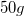of nitrogen gas are contained in a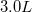container. The gas exerts a pressure of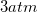on the container.

If pressure is kept constant, what is the final molar amount of gas present in the container if gas is added until the volume has increased to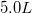?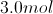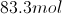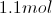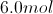Explanation:

Since pressure is kept constant, we can directly compare the moles of gas in the container and volume using Avogadro's law. Since moles of gas and volume are on opposite sides of the ideal gas law, the two variables are directly proportional to one another.

Avogadro's law is written as follows: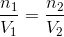First, we will need to convert the initial mass of gas to moles. It is important to remember that nitrogen gas is diatomic!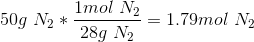Use this value and the given volumes to solve for the final amount of gas in the container.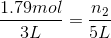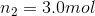### Example Question #1 : Using Avogadro's Law

If 1.0mol of helium gas (He) at standard temperature and pressure (STP) has a volume of 22.4L, how many moles of carbon tetrachloride gas (CCl4) will be present in a container with a volume of 22.4L?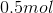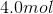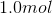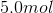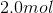Explanation:

Avogadro's Law states that two gases at the same temperature and volume will have an equal number of molecules, and therefore the same number of moles.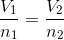It does not matter that helium has one atom per molecule while carbon tetrachloride has five atoms per molecule. Note that the given volume per mole of gas at STP is standard, and true for any gas. At STP, one mole of gas will always have a volume of 22.5L, regardless of its identity.

### Example Question #3 : Using Avogadro's Law

How many neutrons are in 10 moles of aluminum?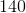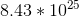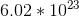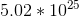Explanation:

To find the number of neutrons, we must convert the moles to atoms by multiplying the moles by Avogadro's number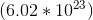.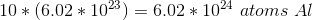Now looking at the periodic table, the neutrons per atom can be found by subtracting the atomic weight by the atomic number: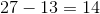Thus there are 14 neutrons in each atom of aluminum.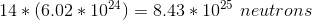### All High School Chemistry Resources